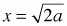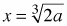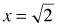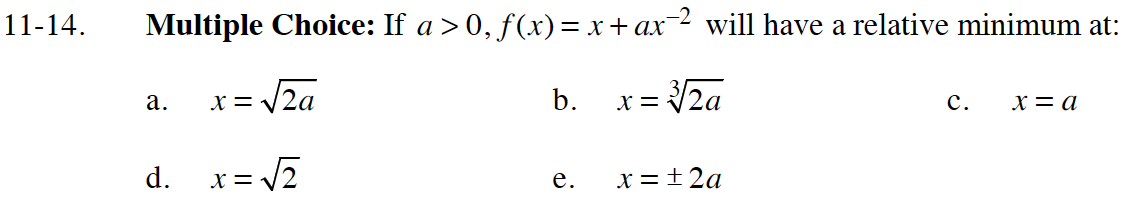### Home > CALC > Chapter 11 > Lesson 11.1.1 > Problem11-14

11-14.
1. Multiple Choice: If a > 0, f(x) = x + ax−2 will have a relative minimum at: Homework Help ✎

1.2.3. x = a

4.5. x = ± 2a$f^\prime(x)=1-2ax^{-3}=0$